# SBI CLERK PRELIMS-REASONING DAY-12

Dear Bankersdaily Aspirants,

SBI Clerk Prelims 2018, will be going to held on March/April (Tentatively) 2018 as per SBI Clerk Notification. This Exam is going to be one of the toughest exam of the year 2018. Because SBI will be the game changer for all the upcoming bank exams. As per as the new pattern is concerned there will be sectional timing for three section in 1 hour. So as per the latest pattern each section is allotted with the sectional timing of 20 minutes.

NEW PATTERN:

Preliminary Examination:Phase I

Phase-I : Preliminary Examination: Preliminary Examination (online) consisting of Objective Tests for 100 marks will be conducted online. This test would be of 1 hour duration consisting of 3 Sections as follows:

<<>>

 SL. Name of test No. of Questions Marks Duration 1. English Language 30 30 20 Minutes 2. Numerical Ability 35 35 20 Minutes 3. Reasoning Ability 35 35 20 Minutes Total 100 100 1 Hour

So start your preparation now with this study Planner for SBI Clerk Prelims 2018. It’s a 30+ days complete planner with various Aptitude, Reasoning, English, topics Included.

Though the exam is getting tougher and tougher for each and every Year, Only consistently hard working candidates will crack the exam. So be a continuous hard worker and grab the opportunity to achieve your success.

SBI Clerk Prelims 2018 Study Planner Day-12

Reasoning-Mathematical Operations-2

Time: 20 Minutes

Can you take this Quiz in 20 minutes?

If you can then attend the quiz. Share your score in our Telegram Group.

You can join in our Telegram Channel the invite given below and you can share this with your friends and other aspirants who are preparing for their exams like you.

Caution: You should have Telegram app installed in your mobile or laptop to join the group. If you don’t have telegram app in your mobile. First install the app and click the link given below….

D.1-5):In the following questions, the symbols @, %, #, \$ and * are used with different meanings explained below. Read the statements carefully and answer the following questions:

P @ Q means P is neither smaller than nor equal to Q.

P % Q means P is neither greater than nor equal to Q.

P * Q means P is neither greater than nor smaller than Q.

P # Q means P is not greater than Q.

P \$ Q means P is not smaller than Q.

1.If the following conclusions O % B and C \$ E are definitely true, then which of following statement is logically satisfies the conclusions?

a) E \$ P @ B* N @ C % O *Y

b) O % Y % N @ B % C \$ P * E

c) Y \$ O % N * B # C \$ P * E

d) E * O * Y % N @ C @ B *P

e) None of these.

2.If the following conclusions A % E and D @ G are should always true, then which of the following statement is logically satisfies the conclusions?

a) A # C \$ D # E # F * G % H

b) H * C * E % F # D @ A % G

c) E @ C * F % H @ A % D * G

d) A # C * F % E \$ D * H @ G

e) None of these.

3.If the following conclusions Z % Y and W # V should always true, then which of the following statement is logically satisfies the conclusions?

a) None of these.

b) Z \$ K # R * I # W % Y @ U * V

c) W % Z % R * U % K \$ I *V % Y

d) V * Z % U @ R % W \$ I * K @ Y

e) K @ Z % R * Y \$ W # I * V @ U

4.In the following conclusions T # S should be false and S \$ P should be true. Then which of the following statement is logically satisfies the conclusions?

a) S @ O % P * Q #G \$ R @ T # V

b) P \$ O % T * Q \$ V \$ R % H * S

c) R* O# S @ G # Q # V @ T * P

d) G @ O % T * Q \$ S \$ R * P # V

e) None of these.

5.In the following conclusions, C @ N should be false and N \$ G should be true. Then which of the following statement is logically satisfies the conclusions?

a) N % A % L * C \$ D @ G * R # M

b) C @ L * A @ G \$ D * M @ R * N

c) C @ L @ A % N \$ D \$ G * R # M

d) C * L @ A % G \$ D % R @ M @ N

e) None of these

D.6-10): Study the following information carefully and answer the given questions.

a) Only I is true

b) Only II is true

c) Either I or II are true

d) Neither I nor II is true

e) Both I and II are true

6.Statements:

P<L≤O=N; L= Q

Conclusions:

N>P,

Q≤O

7.Statements:

Z>Y=X≥N<U≤H; U>P;

Conclusions:

I.H<P

Z < N

8.Statements:

B≥E≥A>C≤A≤F

Conclusions:

B ≥ A

E ≤ F

9.Statements:

X<Z≤A=N≥V; W≥N<O; E≥Z

Conclusions:

Z≤V

X<W

10.Statements:

B<L≤C=M≥D; W≥M<O; F≥L

Conclusions:

W≥D

F<O

D.11-15): Study the following information carefully and answer the given questions.

11.Statements:

P<W=L<F, K>H>M<P, F≤D=N>C

Conclusions:

W>H

N>P

M<F

L<C

a) Only conclusion I is true

b) Only conclusion II is true

c) Only conclusions II and III are true

d) Only conclusions II and IV are true

e) None of these

12.Statement:

L>I=F>U, Y<A≤M>D, C>A≥E=I>W

Conclusion:

I≤M

W<D

Y<F

C>L

a) Only conclusion I and II are true

b) Only conclusion I and IV is true

c) Only conclusion III and I are true

d) Only conclusion II and IV are true

e) None of these

13.Statements:

S>L≥M=K, A=N<J≤S<H, U>F≤X<J

Conclusions:

K≥A

A<K

S>X

H>F

a) Only conclusion I and II are true

b) Only conclusion III and II are true

c) Only conclusion III and IV are true

d) Only IV is true

e) None of these

14.Statements:

H>W≥E=X, V<S≤E=D<F, T≤W>N≥A

Conclusions:

V<H

T≤D

X≥S

D<T

a) Either II or IV follows

b) Only I, III and either II or IV follow

c) Only I and III follow

d) All are true

e) None of these

15.Statements:

D<V=G≤F>S, R≥T>G≤U=W, Z>K≥Y=T>I<O

Conclusions:

K≥U

V<R

D≥I

I>D

a) Only II follows

b) Only IV follows

c) Only II and III follows

d) Only II and either III or IV follow

e) None of these

D.16-20):In the following questions, the symbols >, <, -, \$ and = are used with different meanings explained below. Study the following information carefully and answer the given questions.

‘K\$L’ means ‘K is neither greater than nor equal to L’

‘K>L’ means ‘K is neither smaller than nor equal to L’

‘K<L’ means ‘K is not greater than L’

‘K –L’ means ‘K is neither greater than nor smaller than L’

‘K=L’ means ‘K is not smaller than L’

16.In which of the following statements ‘G\$Z’ and ‘P>F’ is definitely true?

a) Z=P-H>G>F

b) Z=P-G<H<F

c) Z=P=G-H>F

d) Z=P-G\$H<F

e) None of these

17.In which of the following statements ‘D>K’ and ‘S\$G’ is definitely true?

a) G\$D>F-K\$S

b) F\$D>S-K>G

c) K\$S\$D-F\$G

d) K\$F\$S<D-G

e) None of these

18.In which of the following statements ‘Q\$R’ and ‘W\$R’ is definitely true?

a) Q-W\$E>R=T

b) R=W<E-T=Q

c) T\$Q\$E>W-R

d) T\$E\$Q-R\$W

e) None of these

19.In which of the following statements ‘R<C’ and ‘K=D’ is definitely true?

a) L<C\$D-R>K

b) R=K>C\$L-D

c) C=D-R<L<K

d) K>L=C-D\$R

e) None of these

20.In which of the following statements ‘G<H’ and ‘I>J’ is definitely true?

a) G<K-J\$H\$I

b) H-K=G>J\$I

c) G\$K-J=H>I

d) I>H=J-K>G

e) None of these

D.21-25):Study the following questions carefully and answer the given questions.

21.Statements:

X < G ≤ H,   U<H ≤W, A<G

Conclusions:

H ≥ A

U < X

a) Only I follow

b) Either I or II follows

c) Only II follows

d) Both I and II follow

e) Neither I nor II follows

22.Statements:

K > V ≥ N, W ≤ E <P, V> W ≥ C

Conclusions:

P > V

C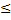E

a) Both I and II follow

b) Neither I nor II follows

c) Either I or II follows

d) Only II follows

e) Only I follow

23.Statements:

S ≥ R >T =E,         W<X ≤R,     U ≤T>M

Conclusions:

X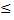M

U < W

a) Neither I nor II follows

b) Only I follow

c) Either I or II follows

d) Only II follows

e) Both I and II follow

24.Statements:

Z < K ≤ H >B,       M > Q ≥ H,           P ≥ Q = T

Conclusions:

Z < Q

P ≥ K

a) Neither I nor II follows

b) Only I follow

c) Both I and II follow

d) Only II follows

e) Either I or II follows

25.Statements:

G < Y ≤ R,   B> V ≥ Y,     D> G ≥L

Conclusions:

V > L

R < D

a) Either I or II follows

b) Only I follow

c) Neither I nor II follows

d) Only II follows

e) Both I and II follow

Explanation:

D.1-5):

 @ > # ≤ % < \$ ≥ * =

1.(c)

Y ≥ O < N = B C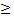P= E

2.(d)

A ≤ C = F < E ≥ D = H > G

3.(e)

K > Z < R = Y ≥ W ≤ I = V > U

4.(d)

G > O < T = Q ≥ S ≥ R = P  V

5.(c)

C > L > A < N ≥ D ≥ G = R  M

6.(e)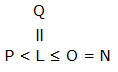7.(d)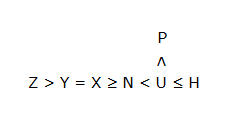8.(a)

B ≥ E ≥ A > C ≤ A ≤ F

9.(b)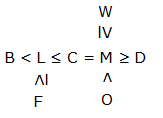10.(a)11.(c)

K > H > M < P < W = L < F ≤ D = N > C

Conclusion:

W > H (False)

N > P (True)

M < F     (True)

L <C (False)

12.(e)

Statement: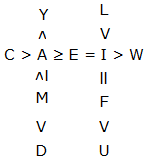Conclusion:

I ≤ M (True)

W < D (False)

Y < F       (False)

C > L (False)

13.(c)

Statement: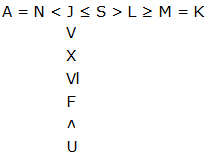Conclusion:

K ≥ A (False)

A < K (False)

S > X     (True)

H < F (True)

14.(b)

Statement: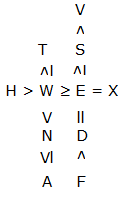Conclusion:

V < H (True)

T ≤ D (False) X ≥ S     (True)

D < T (False)

15.(d)

Statement: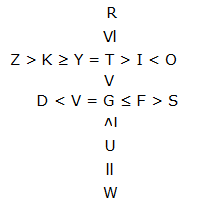Conclusion:

K ≥ U (False)

V < R (True)

D ≥ I      (False)

I > D (False)

D.16-20):

\$ <

>>

< ≤

–  =

=  ≥

16.(a)

Conclusion:

G < Z, P > F

Statement:

Z ≥ P = H > G > F

17.(c)

Conclusion:

D > K, S < G

Statement:

K < S < D = F < G

18.(e)

19.(c)

Conclusion:

R ≤ C, K ≥ D

Statement:

C ≥ D = R ≤ L ≤ K

20.(b)

Conclusion:

G ≤ H, I > J

Statement:

H = K ≥ G > J < I

21.(e)

H ≥ G > A;  U < H ≥ G > X

H ≥ A (False)

U < X (False)

22.(d)

P > E ≥ W < V;     E ≥ W ≥ C

P > V (False)

C ≤ E (False)

23.(a)

X ≤ R > T > M;      U ≤ T < R ≥ X > W

X ≤ M (False)

U < W (False)

24.(c)

Z < K ≤ H ≤ Q ≤ P

Z < Q (True)

P ≥ K (True)

25.(b)

V ≥ Y > G ≥ L;      R ≥ Y > G < D

V > L (True)

R < D (False)

You may also line: